Completion of Figures

Completion of Figures is finding the missing part of figure from the given options, so that the option when put at the missing place completes the figure.

Different types of questions covered in this section are follows

1. Rotational Symmetry Through an Angle of 90°
2. Diagonally Opposite Parts are Similar
3. Adjacent Parts are Laterally / Vertically Inverted Images
4. Based on Certain Design and Pattern

Questions based on completion of figure are divided into two parts, question figure and answer figures. Question figure given on the left side with the answer figures given on the right side. The question figure is incomplete as its one part ( generally 1/4 the figure) is missing denote by the question mark (?). Here, the candidate is required to choose a figure out of the given answer figures that fits in the place of question mark (?) in the same form as it is given to complete the design of the given question figure.

TIPS on cracking Non Verbal Reasoning Questions on Completion of Figures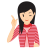Few Important things to Remember

Time is a very important thing in every exams. You should complete your exam within the time frame. But in a competitive exam, question are for testing your calculation ability within a given time frame. They tests, how quickly a student can solve a question paper. This is the reason so many students couldn’t finish their paper within given time. But if you need to solve your government or bank or any other question paper quickly then you should use tips of reasoning and Completion of Figures (Non Verbal Reasoning) which will help you to solve government, bank and other exam papers quickly.

Different questions on completion of figures can broadly be classified into four different types, which are explained as follows

Type - 1 : Rotational Symmetry Through an Angle of 90°

Tip# : In this type of questions, the question figure looks the same if it is rotated by 90° in clockwise or anti-clockwise direction.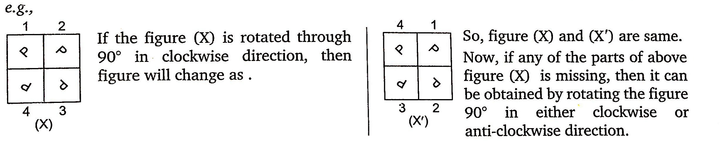Example -1 Identify the missing part of the given figure and select it from given answer figures.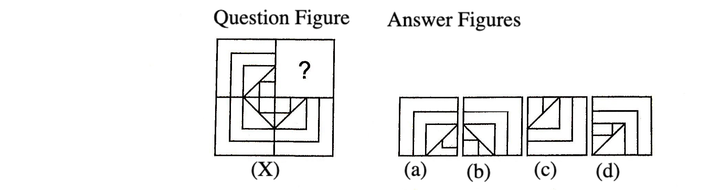Solution: (b) Here, all the three equal parts have similar design, if rotated in clockwise direction. So, we can obtain the answer figure for the missing portion by rotating the question figure by 90° clockwise.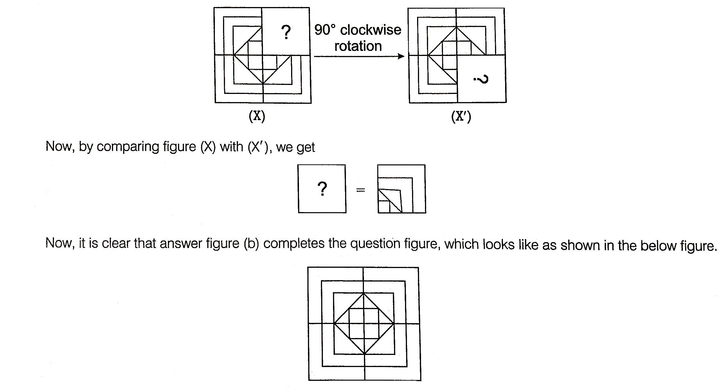Example -2 Identify the missing part of the given figure and select it from given answer figures.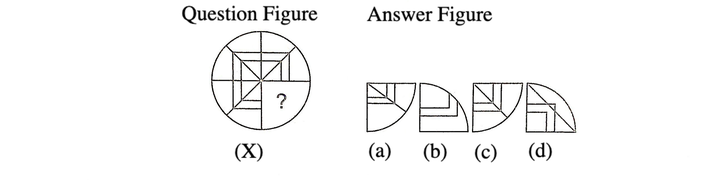Solution: (c) Here, all the three equal parts have similar design, if rotated in clockwise direction. So, we can obtain the answer figure for the missing portion by rotating the question figure by 90° clockwise.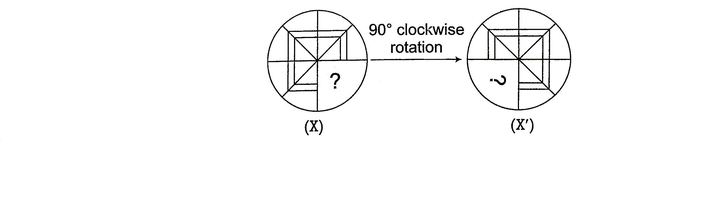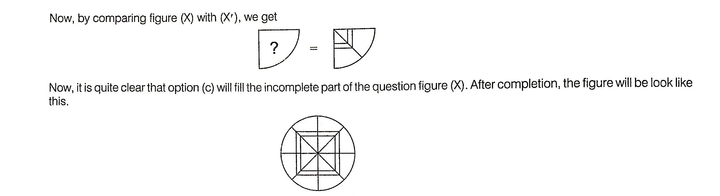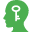Memory Add-ons

1. In some of the questions, the correct option for the missing figure can be given in any rotated from, so candidate can rotate the figures to clock the correctness of option.
2. If answer figures contain similar figure but in rotated forms, then the correct answer figure is that figure which can be substituted at the missing part with least change in orientation.

Example - 3 Which of the answer figures given will complete the pattern of the question figure?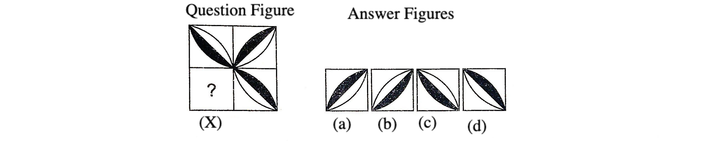Solution: (b) Here, all the three equal parts have similar design, if rotated in clockwise direction. So, we can obtain the answer figure for the missing portion by rotating the question figure (X) by 90° in clockwise direction.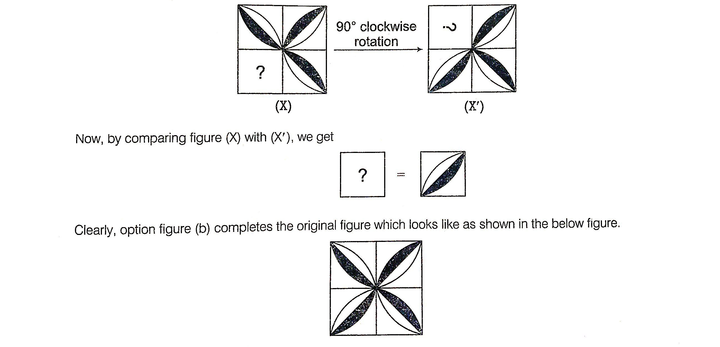Example - 4 In the following question, complete the missing portion of the question figure by selecting from the given answer figures (a), (b), (c) and (d).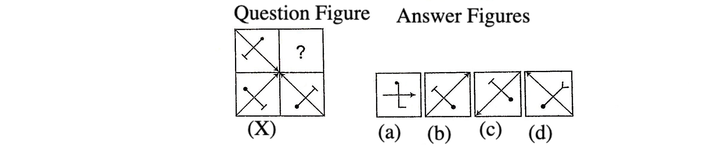Solution: (c) Here, all the three equal parts have similar design, if rotated in clockwise direction. So, we can obtain the answer figure for the missing portion by rotating the question figure (X) by rotating the original given figure by 90° clockwise.Now, it is clear that option (c) will replace (?) to make the figure complete.

Type - 2 : Diagonally Opposite Parts are Similar

Tip# : In this type of questions, the diagonally opposite parts have similar design and if the figure is rotated through 180° in clockwise/anti-clockwise direction the figure remains the same.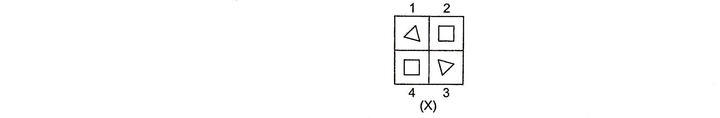In figure (X), diagonally opposite parts i.e., 1-3 and 2-4 have similar design and figure (X) is rotated through 180° , then figure will change as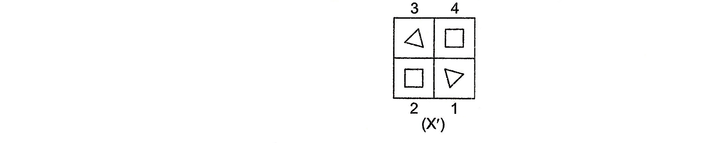So, figures (X) and (X') are same.

Now, if any of the parts of above figure (X) is missing, then it can be obtained by rotating the figure 180° in clockwise/anti-clockwise direction.

This can be better understood with the help of following examples

Example - 1: Identify the missing part of the question figure and select it from given answer figures.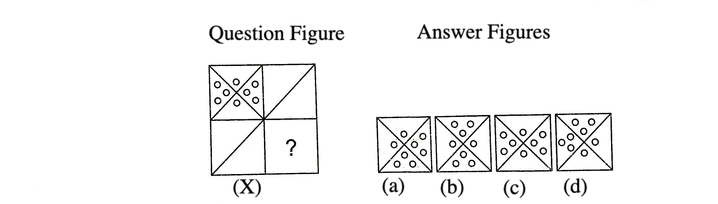Solution: (c) Here, the diagonally opposite parts have similar design.

So, we can obtain the answer figure for the missing portion by rotating the question figure by 180°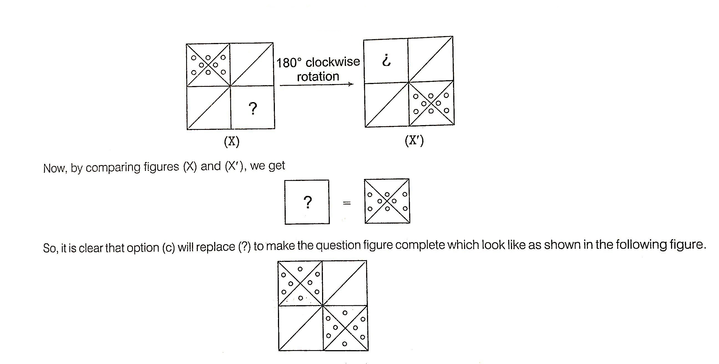Example - 2: Identify the missing part of the question figure and select it from given answer figures.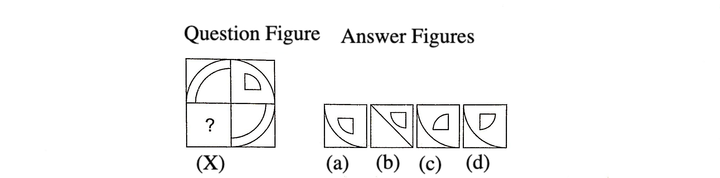Solution: (a) Here, the diagonally opposite parts have similar design.

So, we can obtain the answer figure for the missing portion by rotating the question figure by 180°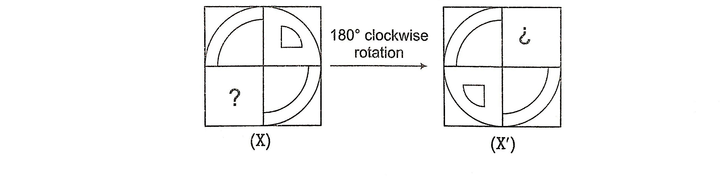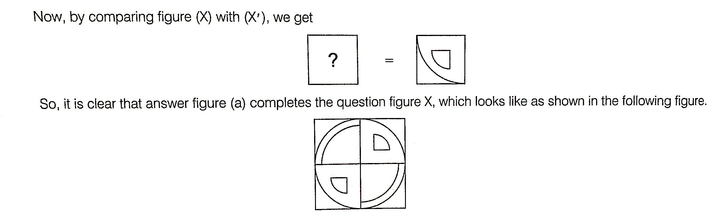Type - 3 : Adjacent Parts are Laterally/Vertically Inverted Images

Tip# : In this type of questions, the adjacent parts are laterally/vertically inverted images of each other.

e.g.,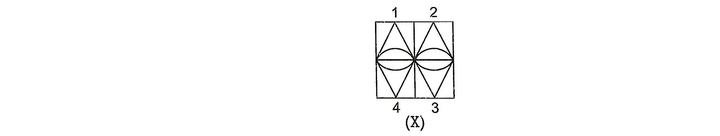In figure (X), 1 and 2 are laterally inverted images of each other. Similarly, 3 and 4 are laterally inverted image of each other. Also 1-4 and 2-3 are the pairs of vertically inverted images of each other.

If any part of above figure is missing, then it found out by the vertical or lateral inversion of any of the adjacent figure.

This can be better understood with the help of following example

Example - 1: Identify the missing part of the question figure and select it from given answer figures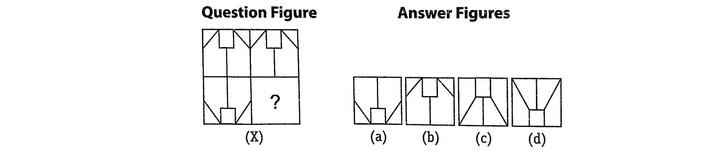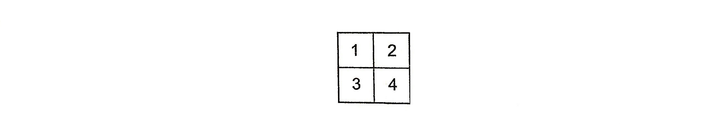Solution: (a) Here, the upper two adjacent parts i.e., (1) and (2) have design which are laterally inverted form of each other and the two left side adjacent parts i.e., (1) and (3) have designs which are vertically inverted form of each other. Then, the design in missing part i.e., (4) will be the laterally inverted form of the design in part (3). So, from the answer options given it is clear that design given in option (a) is the correct laterally of (3) and it will replace the (?) to complete the pattern.

Example - 2: Identify the missing part of the question figure and select it from given answer figures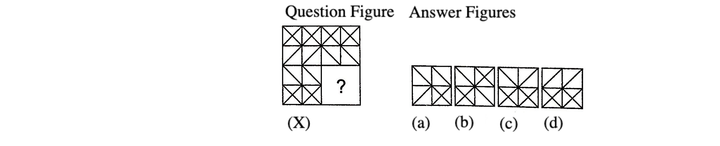Solution: (d) Let the figure isHere, the upper two adjacent parts i.e., (1) and (2) have design which are laterally inverted form of each other. Then, the design in missing part i.e., (4) will be the laterally inverted form of the design in part (3). So. from the answer options given it is clear that design given in option (d) is the correct laterally inverted form of (3) and replace the (?) to complete the pattern.

Type - 4 : Based on Certain Design and Pattern

Tip# : In this type of questions, they do not follow any of the patterns discussed in the previous types. These questions contain a certain design or pattern with one of its parts missing. A candidate has to complete the design accordingly.

This can be better understood with the help of following examples

Example - 1: Identify the missing part of the question figure and select it from the answer figures.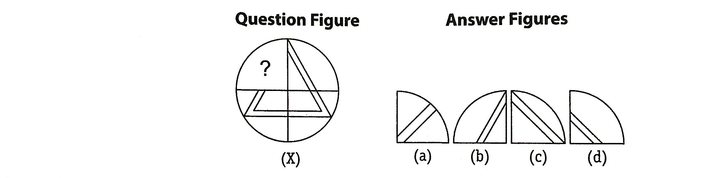Solution: (b) Here, by analysis we can observe that the given pattern is of a doubled lined triangle.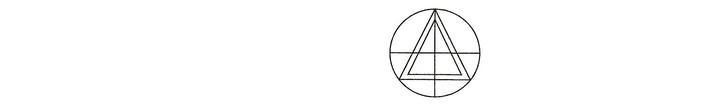Example - 2: Identify the missing part of the question figure and select it from the answer figures.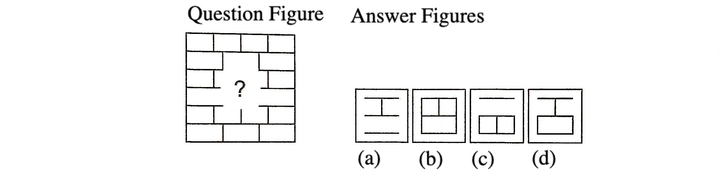Solution: (d) Here, by analysis we can observe that in the figure the design of each line repeats itself in alternate lines.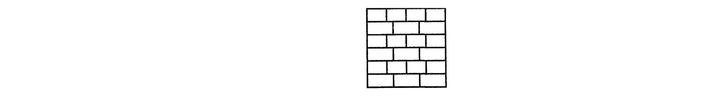LearnFrenzy provides you lots of fully solved Completion of Figures Questions and Answers with explanation.